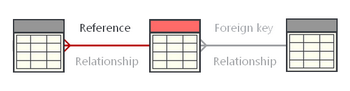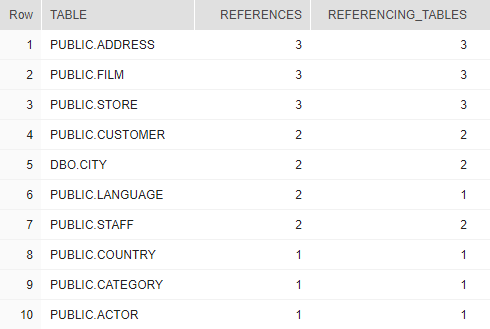## List most referenced tables (by FK) in Snowflake

Article for:

Query below allow to obtain list of most referenced tables by foreign keys.## Query

``````select pk_tco.table_schema || '.' || pk_tco.table_name as "TABLE",
count(*) as references,
count(distinct fk_tco.table_name) as referencing_tables
from information_schema.referential_constraints rco
join information_schema.table_constraints fk_tco
on rco.constraint_name = fk_tco.constraint_name
and rco.constraint_schema = fk_tco.table_schema
join information_schema.table_constraints pk_tco
on rco.unique_constraint_name = pk_tco.constraint_name
and rco.unique_constraint_schema = pk_tco.table_schema
group by "TABLE"
order by count(*) desc;
``````

## Columns

• table - table name preced by schema name
• references - number of references to this table
• referencing_tables - number of tables which are referencing to this table (single table can have multiple foreign keys referencing to this table)

## Rows

• One row represents one table
• Scope of rows: all tables referenced by foreign keys
• Ordered by number of references descending

## Sample results

Notice that PUBLIC.LANGUAGE table is referenced 2 times from one table.